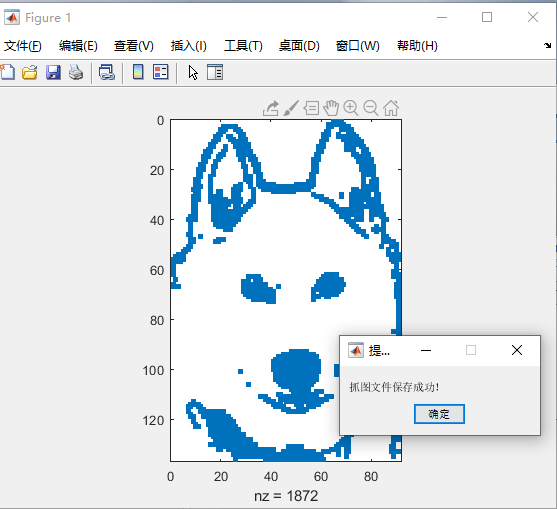• MATLAB截屏

2019-03-11 12:46:45
MATLAB代码实现截屏功能，直接运行即可，如需存储可自行存储MATLAB
• MATLAB截图

2021-04-26 14:44:45
MATLAB截图1.[代码][其他]代码function screen_capture(movie_name,recording_time)%Can be used to record any screen activity and output%the recorded actions as video file (MOVIE.avi). It also saves the ...

MATLAB截图

1.[代码][其他]代码

function screen_capture(movie_name,recording_time)

%Can be used to record any screen activity and output

%the recorded actions as video file (MOVIE.avi). It also saves the frames used to

%create the movie in a jpg format frames. The images are named as

%scrcapture -frame number- .jpg

%For example the first frame is named scrcapture1.jpg

%The recording time is almost identical to the actual cpu time.

%The quality of the video is excellent, but the size is big. One is advised

%to record short videos(order of minutes).For even better quality, one could replace the

% png format to jpg(simply uncomment the respective commands).

% to call the function, one types:

%

% screen_capture(movie_name,recording_time)

% where

% movie_name: is a string representing the name of the movie

% recording_time: is the length of the capturing (in seconds)

%To try, one might use the following example:

% screen_capture('movie',30)

%When the code is done writing the movie, a gong sound will be played

%to inform the user that the movie is finished.

%%%%%%%%%%%%%%%%%%%%%%%%%%%%%%%%%

%This function is written by :

% Nassim Khaled

% Wayne State University

% Phd Candidate

%%%%%%%%%%%%%%%%%%%%%%%%%%%%%%%%

movie_name1=strcat(movie_name,'.avi')

mov = avifile(movie_name1);

count=0;

robo = java.awt.Robot;

t = java.awt.Toolkit.getDefaultToolkit();

rectangle = java.awt.Rectangle(t.getScreenSize());

number_of_frames=round(recording_time/0.65);

display_time_of_frame=10;

for i=1:number_of_frames

name1=strcat('scrcapture',num2str(i),'.png');

% name1=strcat('scrcapture',num2str(i),'.jpg');

image = robo.createScreenCapture(rectangle);

filehandle = java.io.File(name1);

javax.imageio.ImageIO.write(image,'png',filehandle);

% javax.imageio.ImageIO.write(image,'jpg',filehandle);

end

for i=1:number_of_frames

name1=strcat('scrcapture',num2str(i),'.png');

% name1=strcat('scrcapture',num2str(i),'.jpg');

while count

count=count+1;

F = im2frame(a);

end

count=0;

end

close all

mov=close(mov);

% wavplay(y,Fs)

展开全文• Matlab中实现截图功能。主要调用的自带的JavaMatlab 截屏
• spy%产生matlab 狗 图像 f=getframe(gcf); f=frame2im(f); imwrite(f,"11.bmp"); msgbox('抓图文件保存成功！', '提示信息'); 也可以采用下面这个函数，可以在你的GUI中作为截图的callback函数。 function ...

写作时间：2020-08-05
目录：

正文:代码：
示例如下：

spy%产生matlab 狗 图像
f=getframe(gcf);
f=frame2im(f);
imwrite(f,"11.bmp");
msgbox('抓图文件保存成功！', '提示信息');

也可以采用下面这个函数，可以在你的GUI中作为截图的callback函数。

function snapImage_func()
% ********************************************************
% Filename    : snapImage_func.m
% Author      : CSDN Mario图像算法
% Description : 在matlab 中截图
% Revision    : 2020/11/04
% *********************************************************
currentNewPathname = fullfile(pwd, 'snap_images');
%pwd  确定当前文件夹
if ~exist(currentNewPathname, 'dir')
mkdir(currentNewPathname);
end

[filename,pathname,~] = uiputfile({'*.jpg;*.tif;*.png;*.gif','All Image Files';...
'*.*','All Files' },'保存截图',...
fullfile(pwd, 'snap_images\\temp.jpg'));
if isequal(filename, 0) || isequal(pathname, 0)
warndlg('路径不对！', '提示信息');%创建警告对话框
return;
end
pathfilename= fullfile(pathname,filename);
f=getframe(gcf);
f=frame2im(f);
imwrite(f, pathfilename);
msgbox('抓图文件保存成功！', '提示信息');
end

THE END~

展开全文• matlab截图

2015-03-26 22:17:47
利用matlab对图片进行截图，利用简单的命令对图标进行截图matlab
• %-----------------------------...% Matlab截图程序 %---------------------------------------- [filename, pathname] = uigetfile({’.jpg’; '.bmp’; ‘.gif’; '.png’ }, ‘选择图片’); %没有图像 if fi...

%----------------------------------------
%                Matlab截图程序
%----------------------------------------
[filename, pathname] = uigetfile({'*.jpg'; '*.bmp'; '*.gif'; '*.png' }, '选择图片');
%没有图像
if filename == 0
return;
end
[m, n, z] = size(src);
figure(1)
imshow(src)%显示原图像
%----------------------------------------
%拷贝选取图片
%%固定窗口大小
imcrop( src, [265,95,679,579] );%imcrop的参数为[265,95,679,579]，
%代表左上角坐标为[265,95]，裁剪的长度为679，宽度为579
imwrite(imCpR,strcat('E:\experiment\BPNN_edge\moreFrameImg\allPic\imCp\','imCpR','.bmp'),'bmp');
figure(2)
imshow(imCpR);

展开全文• 百度文库-让每个人平等地提升自我9matlab截图到期刊论文中如何保持清晰度的方法一、总的介绍

百度文库-让每个人平等地提升自我

9matlab截图到期刊论文中如何保持清晰度的方法一、总的介绍

展开全文• 截图首先要进行的就是选取你要截图的正方形的点，选取方式，调用函数：[x,y,c]=ginput(n);即可。上面的函数中n参数用于决定图像中选取的点的个数，x，y返回的是所选取的点的坐标，c返回的是你选择的键的类型：是左键...
• 国外写的一个比较好的matlab截图代码，使用很简单，解压出来即可使用。
• Matlab屏幕截图终极总结

万次阅读 2018-03-15 09:48:50
%%%%%-Matlab截取屏幕-%%%%%%%%%%%%%%%%%%%%%%%%%%%%%%%%%%%%%%%%%%%%%%%%%%%%%%% robot = java.awt.Robot(); %创建java.awt.Robot对象robot，自动测试 rectangle = java.awt.Rectangle(); %创建java.awt.Rectangle...Matlab
• MATLAB截取屏幕图像进行处理的代码，是调用了java的控件，可以将桌面上的图像截取，方便后续的处理。 代码直接可以在MATLAB上运行
• 执行上述代码时需要注意，选择合适的矩形框完成截图时，应该在框内双击左键，便完成了手动选择区域的截图。 之后，我们可以在工作区域看到生成一个rect，表示截图成功。 对手动截图后的图像进行显示。 figure(2),...matlab
• 代码：%%%img_path:图像所在文件夹%save_path：裁剪后的图像保存文件夹%双击左键不截图，跳到下一张%%img_path='E:\image\';save_path='E:\image\';name_beg=0;%截取的图像保存名字从0开始Files = dir(img_path);for...
• 用MATLAB实现屏幕截屏的代码

千次阅读 2014-12-04 16:11:36
function screen_capture(movie_name,recording_time) %Can be used to record any screen activity and output %the recorded actions as video file (MOVIE.avi). It also saves the frames used to ...
• MATLAB实现截屏的小程序

热门讨论 2009-08-28 11:45:15
解压之后可以直接用MATLAB轻松实现截屏，一个小小的程序，可以帮我们不少忙MATLAB小程序
• 下面是活动activeline效果，请注意它与上面的屏幕截图有何不同。 如果indentBase为dontchange ，则dontchange -align将仅对齐具有相同缩进的行，并且不会修改该缩进。 matlab-align.surroundSpace 默认值： matlab-...
• matlab代码对齐星型对齐 通过图像中的星点对齐多个天文学/夜景图像。 适用于广角镜和远摄镜。 有关动机和算法的详细博客，请参见（这是我在zhihu.com上的专栏，当然是中文） Matlab代码 检查star_align_average_main...
• 此示例向您展示了如何使用 MATLAB 控制安捷伦示波器、捕获波形并在仪器上抓取屏幕截图。 用户可以自定义代码，以允许使用不同的段数点，采样率等。有关用于控制仪器的SCPI命令的更多信息，请参阅仪器的程序员指南。...matlab
• 此示例说明如何使用 MATLAB 和 Instrument Control Toolbox(TM) 软件在仪器显示屏上抓取屏幕截图。 请注意，此示例不会更改 X 系列信号分析仪的设置。 有关用于控制仪器的 SCPI 命令的更多信息，请参阅仪器的程序员...matlab
• 常见的图像变换结果经常带有黑边，如何利用MATLAB有效选择自己需要的图像内容？ 即如何简单提取ROI（region of interest，感兴趣区域） 最近学到了两种简单方法，利用MATLAB中的imcrop函数实现交互式截取ROI,问题就...matlab 图像处理 计算机视觉
• matlab图像截图

2015-04-21 21:06:58
matlab代码，图像截图，修改文件位置，可以直接使用matlab 截图
• matlab中存档算法代码QuestPlus：QUEST +自适应心理测量方法的MATLAB实现 QuestPlus是QUEST +自适应心理测量方法的MATLAB实现。 它提供了一种快速灵活的方法来估算心理物理模型的参数，并且还能够就最合适的刺激以及...
• matlab图像裁剪

2021-04-23 19:04:33
Matlab 图像几何操作_数学_自然科学_专业资料。第2章 图像几何操作 ? 2.1 图像的裁剪、缩放与旋转 ? 2.2 图像的几何变换 ? 2.3 图像的邻域操作 ? 2.4 ......只有迅速掌握 这部分内容,才能开始图象处理的研究 工作。 ...
• MATLAB一些实用的小程序-Matlab.rar 有一些是我自己写的，有一些是网上的，希望对大家有帮助。都是关于线性插值，包括牛顿、Neville，以及微分方程的解法。  共同分享 QQ截图未命名.bmpmatlab
• MATLAB裁剪图片

2021-05-08 12:20:29
考试报名需要上传照片，但是大小比例有要求，高宽比要求为4:3，用MATLAB来进行裁剪。1. 暴力法原图为护照照片，比例为高1080，宽1626，需要将宽变为810，采用逐个值代替法。注意需要将值变为uint8才可以保存成图片并...
• 屏幕截图提供了该过程的图形说明，书面说明如下。 zip 文件中提供了与快捷方式一起使用的图标。 快捷方式创建说明 1. 解压附带的 zip 文件并将图标图像 ExplorerIcon.gif 解压缩到您要存储的位置（例如，c:\MATLAB...matlab
• matlab 进行语音处理，主要有语音端点检测，自相关，基音周期检测，AR系数，语音合成等等，内有一份详细的实验报告，有这个步骤的实验截图及问题分析，在基音周期检测方面，处了用传统的相关法检测外，还用了最近......

matlab截图matlab 订阅